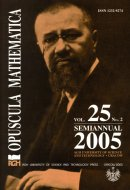Opuscula Math. 25, no. 2 (2005), 275-285

Opuscula Mathematica

# On intertwining and w-hyponormal operators

M. O. Otieno

Abstract. Given $$A, B\in B(H)$$, the algebra of operators on a Hilbert Space $$H$$, define $$\delta_{A,B}: B(H) \to B(H)$$ and $$\Delta_{A,B}: B(H) \to B(H)$$ by $$\delta_{A,B}(X)=AX-XB$$ and $$\Delta_{A,B}(X)=AXB-X$$. In this note, our task is a twofold one. We show firstly that if $$A$$ and $$B^{*}$$ are contractions with $$C_{.}o$$ completely non unitary parts such that $$X \in \ker \Delta_{A,B}$$, then $$X \in \ker \Delta_{A*,B*}$$. Secondly, it is shown that if $$A$$ and $$B^{*}$$ are $$w$$-hyponormal operators such that $$X \in \ker \delta_{A,B}$$ and $$Y \in \ker \delta_{B,A}$$, where $$X$$ and $$Y$$ are quasi-affinities, then $$A$$ and $$B$$ are unitarily equivalent normal operators. A $$w$$-hyponormal operator compactly quasi-similar to an isometry is unitary is also proved.

Keywords: $$w$$-hyponormal operators, contraction operators, quasi-similarity.

Mathematics Subject Classification: 47B20.

Full text (pdf)

• M. O. Otieno
• University of Nairobi, Department of Mathematics, P.O. Box 30197, Nairobi, Kenya